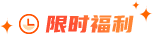### 无重复字符的最长子串题目描述

给定一个字符串，请你找出其中不含有重复字符的 最长子串 的长度。

示例 1:

输入: "abcabcbb"

输出: 3

解释: 因为无重复字符的最长子串是 "abc"，所以其长度为 3.

题目解析

建立一个256位大小的整型数组 freg ，用来建立字符和其出现位置之间的映射。

维护一个滑动窗口，窗口内的都是没有重复的字符，去尽可能的扩大窗口的大小，窗口不停的向右滑动。

(1)如果当前遍历到的字符从未出现过，那么直接扩大右边界;

(2)如果当前遍历到的字符出现过，则缩小窗口(左边索引向右移动)，然后继续观察当前遍历到的字符;

(3)重复(1)(2)，直到左边索引无法再移动;

(4)维护一个结果res，每次用出现过的窗口大小来更新结果 res，最后返回 res 获取结果。

代码实现

// 滑动窗口

// 时间复杂度: O(len(s))

// 空间复杂度: O(len(charset))

class Solution {

public:

int lengthOfLongestSubstring(string s) {

int freq = {0};

int l = 0. r = -1; //滑动窗口为s[l...r]

int res = 0;

// 整个循环从 l == 0; r == -1 这个空窗口开始

// 到l == s.size(); r == s.size()-1 这个空窗口截止

// 在每次循环里逐渐改变窗口, 维护freq, 并记录当前窗口中是否找到了一个新的最优值

while(l < s.size()){

if(r + 1 < s.size() && freq[s[r+1]] == 0){

r++;

freq[s[r]]++;

}else { //r已经到头 || freq[s[r+1]] == 1

freq[s[l]]--;

l++;

}

res = max(res, r-l+1);

}

return res;

}

};### 大数据师资团队更多>>

• 北京校区
• 大连校区
• 广州校区
• 成都校区
• 杭州校区
• 长沙校区
• 合肥校区
• 南京校区
• 上海校区
• 深圳校区
• 武汉校区
• 郑州校区
• 西安校区
• 青岛校区
• 重庆校区
• 太原校区
• 沈阳校区
• 南昌校区
• 哈尔滨校区